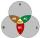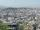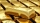# Power + exponential function - problems

1. Profit growthThe profit of a company increased by 25% during the year 1992, increased by 40% during the year 1993, decreased by 20% in the year 1994 and increased by 10% during the year 1995. Find the average growth in the profit level over the four years periods?
2. CrystalCrystal grows every month 1.9 promile of its mass. For how many months to grow a crystal from weight 360 g to 593 g?
3. SubsetsHow many are all subsets of set ??
4. DepositIf you deposit 959 euros the beginning of each year, how much money we have at 2.1% (compound) interest after 19 years?
5. LoanApply for a \$ 59000 loan, the loan repayment period is 8 years, the interest rate 7%. How much should I pay for every month (or every year if paid yearly). Example is for practise geometric progression and/or periodic payment for an annuity.
6. InterestCalculate how much you earn for 10 years 43000 deposit if the interest rate is 1.3% and the interest period is a quarter.
7. To the cinemaJane at 8 am got message that all 1093 school pupils will go to the cinema. Within 20 min she said it to the three friends. Each of them again for 20 minutes said to the other three. In this way the message spread further. At what time all the children in.
8. The city 2Today lives 167000 citizens in city. How many citizens can we expect in 11 years if their annual increase is 1%?
9. The cityAt the end of 2010 the city had 248000 residents. The population increased by 2.5% each year. What is the population at the end of 2013?
10. Exponential equationSolve for x: (4^x):0,5=2/64.
11. DoublingThe message is spreading that each day has doubled the number of people who know about it. All know message for 20 days. How long known it eighth people?
12. Exponential equationFind x, if 625 ^ x = 5 The equation is exponential because the unknown is in the exponential power of 625
13. Geometric progressionIn geometric progression, a1 = 7, q = 5. Find the condition for n to sum first n members is: sn≤217.
14. Precious metalsIn 2006-2009, the value of precious metals changed rapidly. The data in the following table represent the total rate of return (in percentage) for platinum, gold, an silver from 2006 through 2009: Year Platinum Gold Silver 2009 62.7 25.0 56.8 2008 -41.3 4
15. Radioactive materialA radioactive material loses 10% of its mass each year. What proportion will be left there after n=6 years?
16. Investment1000\$ is invested at 10% compound interest. What factor is the capital multiplied by each year? How much will be there after n=12 years?

Do you have an interesting mathematical problem that you can't solve it? Enter it, and we can try to solve it.

To this e-mail address, we will reply solution; solved examples are also published here. Please enter e-mail correctly and check whether you don't have a full mailbox.